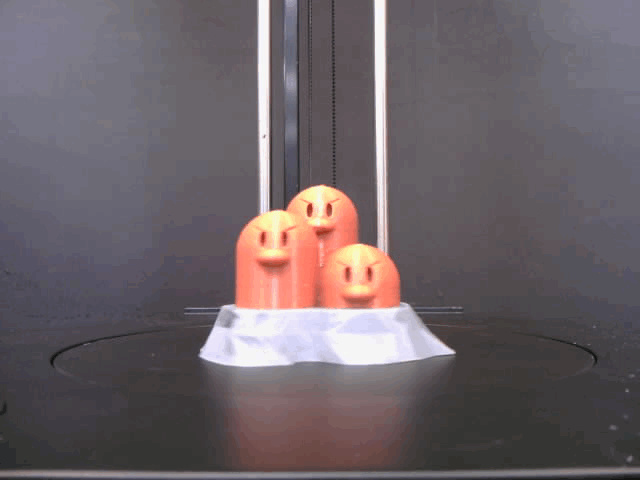# 5.2. Gif Generator¶

This example shows how to generate a gif picture by using scan camera and motor , have fun with FLUX Delta !```# !/usr/bin/env python3

import sys

import imageio  # pip install imageio
import numpy as np

from fluxclient.sdk.delta import Delta

def main():
frames = 180
filename = "my_gif.gif"
images = []

# set blocking flag to true, this will return move() until it actually finish
delta = Delta.connect_delta(ip='192.168.18.114', client_key='./sdk_connection.pem', kick=True, blocking=True)
# move a bit to lock the motor
delta.move(E2=0, speed=2400)

for i in range(frames):
delta.move(E2=360 / frames, relative=True)  # note that we use relative moving here
img = delta.get_image()  # retrieve the image from delta
images.append(img)

delta.close()

# store the gif
images_to_gif(filename, images)

def images_to_gif(filename, images):
imageio.mimsave(filename, [np.array(img) for img in images])

if __name__ == '__main__:
main()
```